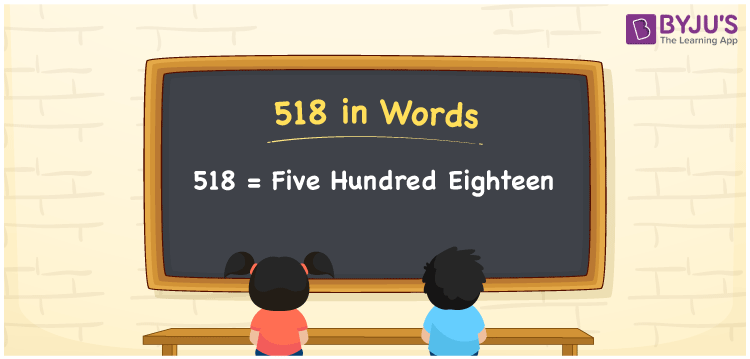# 518 in Words

518 in words is written as Five hundred eighteen. In both the International System of Numerals and the Indian System of Numerals, 518 is written as Five hundred eighteen. The number 518 is a Cardinal Number as it represents some quantity. For example, “518 tables and chairs are required”.

 518 in Words Five hundred eighteen Five hundred eighteen in Number 518

## 518 in English Words

We write 518 in Words using the letters of the English alphabet. Therefore, we read 518 in English as “Five hundred eighteen.”## How to Write 518 in Words?

To write 518 in words, we shall use the place value chart. In the place value chart, write 5 in the hundreds, 1 in the tens, and 8 in the ones, respectively. Now let us make a place value chart to write the number 518 in words.

 Hundreds Tens Ones 5 1 8

Thus, we can write the expanded form as

5 × Hundred + 1 × Ten + 8 × One

= 5 × 100 + 1 × 10 + 8 × 1

= 500 + 10 + 8

= 518

= Five hundred eighteen.

518 is a natural number, the successor of 517 and the predecessor of 519.

518 in words – Five hundred eighteen

• Is 518 an odd number? – No
• Is 518 an even number? – Yes
• Is 518 a perfect square number? – No
• Is 518 a perfect cube number? – No
• Is 518 a prime number? – No
• Is 518 a composite number? – Yes

## Frequently Asked Questions on 518 in Words

Q1

### How to write 518 in words?

518 in words is written as Five hundred eighteen.
Q2

### How to write 518 in the International and Indian System of Numerals?

In both, the system of numerals, 518 in words, is written as Five hundred eighteen.
Q3

### What is the preceding number of 518?

The number that precedes 518 is 517.